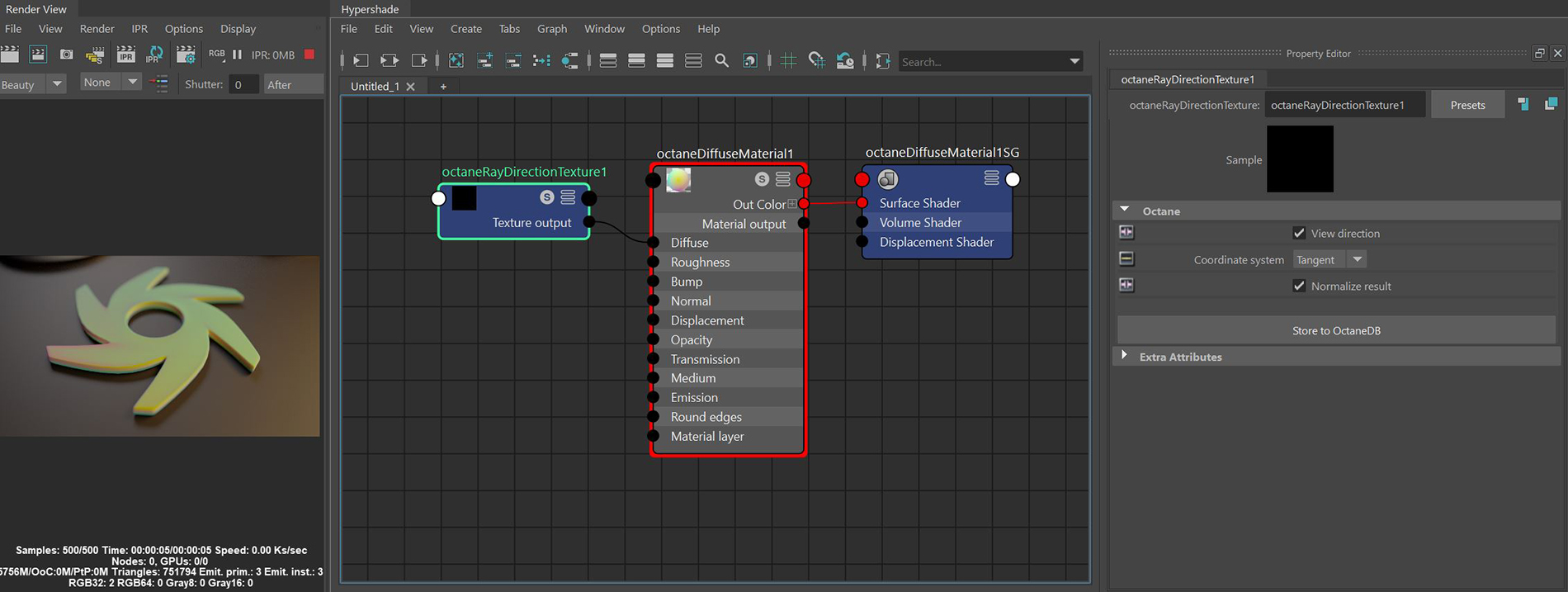# Ray Direction Texture

The Ray Direction node converts the direction of the incoming ray into an RGB texture (figure 1).### Ray Direction Texture Attributes

View Direction - If checked, the resulting vector is determined from the viewing position to the shaded point position, if not checked, the data is calculated in the opposite direction.

Coordinate System - The coordinate space used to output the ray direction.

• World - Coordinates are calculated from the absolute origin of the scene (0,0,0).
• Object - Coordinates are calculated by the orientation of the object on which the node is applied.
• Camera - Coordinates are calculated from the origin of the camera's center.
• Tangent - Coordinates are calculated across the surface of an object where x and y can be thought of as U and V (texture space) and the Z direction is each faces' normal direction.

Normalize Result - Determines whether to rempa the results to the 0 to 1 range or leave it in the -1 to 1 range.